#Interactive Real Analysis

Next | Previous | Glossary | Map

## 4.3. Special Series

### Theorem 4.2.9: Geometric Series

Let a be any real number. Then the series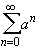is called Geometric Series.
• if | a | < 1 the geometric series converges
• if | a |1 the geometric series diverges
If the geometric series converges (i.e. if | a | < 1) then=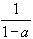Note that the index for the geometric series starts at 0. This is not important for the convergence behavior, but it is important for the resulting limit.

 Examples 4.2.10:Investigate the convergence behavior of the following series: What is the actual limit of the sum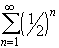? What is the actual limit of the sum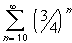? Does the sum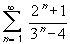converge ? (Here the limit comparison test may be helpful).
Proof:

The proof consists of a nice trick. Consider the partial sum S N and multiply it by a:

• S N = 1 + a + a 2 + a 3 + ... + a N
• a S N = a + a 2 + a 3 + ... + a N+1
Subtracting both equations yields: (1 - a) SN = 1 - a N+1. Dividing both sides by (1 - a) and taking the limit, the result follows from previous result on the power sequence.Next | Previous | Glossary | Map